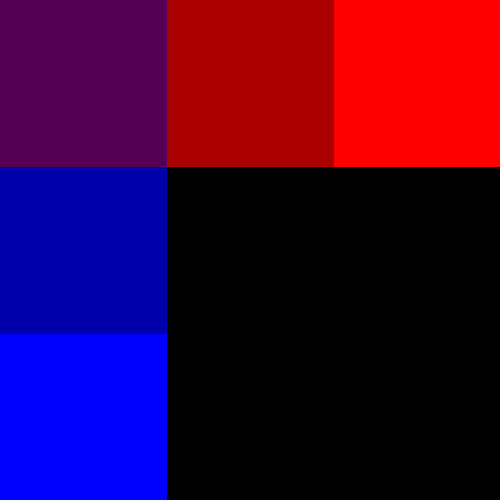# G'MIC

## input

Using the input command (to draw lines and such, at least) proved harder than I expected on the PowerShell Command Line.

Inputing an image, however, was just as easy as I expected.

Input a Single Image

C:\alpha\gmic\gmic.exe input C:\alpha\input\image_in.jpg rotate 180 output C:\alpha\output\image_out.jpg

Although single-liners (per above) are great on the Command Line, I like multi-liners for write-ups like this. The back-tick () at the end is the line continuation symbol for PowerShell.
C:\alpha\gmic\gmic.exe 
input C:\alpha\input\image_in.jpg
rotate 180 
output C:\alpha\output\image_out.jpg

• C:\alpha\gmic\gmic.exe
• G'MIC is not on my path, so I need to call it explicitly.
• input C:\alpha\input\image_in.jpg
• Places the called image on the stack.
• rotate 180
• Does stuff.
• output C:\alpha\output\image_out.jpg
• Exports the last (and in this case, only) image on the stack.
• There are ways to export the entire stack as a sequential list of images, but I'm not covering that here.
• Though, as a first step, I'd try using '1', '01', '#', or '##' in the save name.
• Or one could type gmic help output on the Command Line and that might provide some useful information.

Blank Images

C:\alpha\gmic\gmic.exe input 500,500,1,3

That last part (500,500,1,3) denotes the size of an image to be created, which is initialized full of zeroes (i.e. black).

The lack of an output command causes G'MIC to call display prior to exiting.

The following creates two black images (of different size) and displays them side by side.
C:\alpha\gmic\gmic.exe
input 
500,500,1,3
200,200,1,3

The numbers in order represent Width, Height, Depth, and the Number of Colour Channels (3 for a RGB image). Personally, I find it a bit odd to add the one (for Depth) in there all the time, as Multi-Dimensional images are not my thing. But then, I don't know how these data structures are implemented under the hood, so perhaps it makes perfect sense... which one can only assume it did for G'MIC's creators. Either way, an extraneous one is much less confusing than NumPy's reversal of height and width.

W,H,D,C

{In truth, having a Depth Dimension in there all the time makes sense. Originally, I thought that Depth was Number of Frames. But that is not the case. The images are stored in a list (implicitly). So, that's where the Frames are stored (as sequential images). Meaning, my confusion (if not yours) has been resolved.}

Dot Mapping

The following creates a single-pixel all-purple image.

C:\alpha\gmic\gmic.exe input '(1^0^2)'

Those are RGB colour values between the carats: '(R^G^B)'

Since the colors intensities are relative (there is no absolute scale), all of the following inputs create the same image.

{Note: I am dropping the C:\alpha\gmic\gmic.exe input part for clarity.}
'(.5^0^1)'
'(1^0^2)'
'(128^0^255)'

As stated, if no output is declared, the code ends with an implicit display. But the viewer can be misleading (as it displays a 1px image as a large square, many times larger than a single pixel).

In order to create usable images (unless one is looking for a 1px image) a few more lines of code are required.C:\alpha\gmic\gmic.exe 
input '(128^0^255)'
normalize 0,255 
resize 500,500,1,3
output C:\alpha\output\128_0_255.jpg

More complex Dot Mapping is possible.
• , denotes the next pixel
• ; denotes a new line
• ^ shifts the color channel
• Punctuation is between...
• Not after.
• And lo to thee who gets any of this wrong.
For a 3x3 purplish color grid, the following denotes the dot map.

'(1,2,3;1,2,3;1,2,3^0^1,1,1;2,2,2;3,3,3)'

Please note, empty spaces in a Dot Map are taken to be zero.

Meaning, there is no broadcasting built into G'MIC.WORKS
Creates a Full 3x3 Grid (left image, above)

input '(1,2,3;1,2,3;1,2,3^0^1,1,1;2,2,2;3,3,3)'

  R     G     B

1 2 3   0   1 1 1
1 2 3       2 2 2
1 2 3       3 3 3


DOES NOT WORK
3x3 Grid is Missing 4 Squares (right image, above)

input '(1,2,3^0^1;2;3)'

  R     G     B

1 2 3   0   1
2
3


Though in the end, the definition of 'Working' and 'Not Working' is highly subjective.

Also, I feel the need to reiterate that to save the nine pixel image which has been created in a usable format (which to me means as a much larger image), I had to use the resize command.

C:\alpha\gmic\gmic.exe 
input '(1,2,3;1,2,3;1,2,3^0^1,1,1;2,2,2;3,3,3)'
-normalize 0,255 
-resize 500,500,1,3
output C:\alpha\output\grid_full.png


{And just now, I am noticing the subtle difference in the colours between the two images. I am going to assume (so, I could be wrong) it comes from the difference in normalizing 1,2,3 versus normalizing 0,1,2,3 to the range 0-255.}

Formulas

Rather than creating a zeroed out image, one can insert a formula at the end of the constructor.

gmic input 500,500,1,3,'y=x'

There are no spaces between the arguments.

Width,Height,Depth,Colour,Formula are all smashed together.

W,H,D,C,'x'

'y=x' creates a black and white ramp... call it a gradient.

Originally, it was my intent to explore what could be accomplished procedurally with G'MIC (i.e. by using formulas). But in the end, I believe it will be easier to input images created elsewhere (such as in InkScape) or simply using one of the pre-made images available via the clut command. So, I'm not going to spend anymore time trying to create lines, circles, or spherical multi-colored ramps... at least, not at this particular juncture in time.